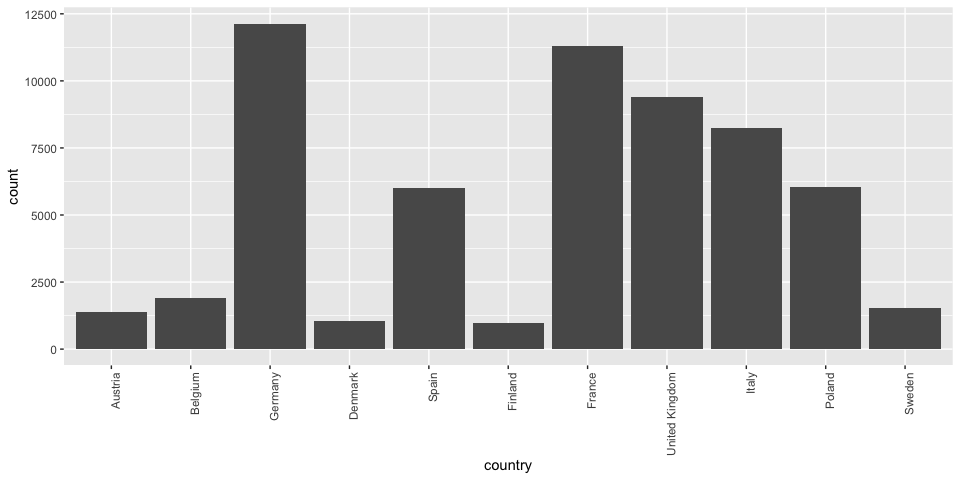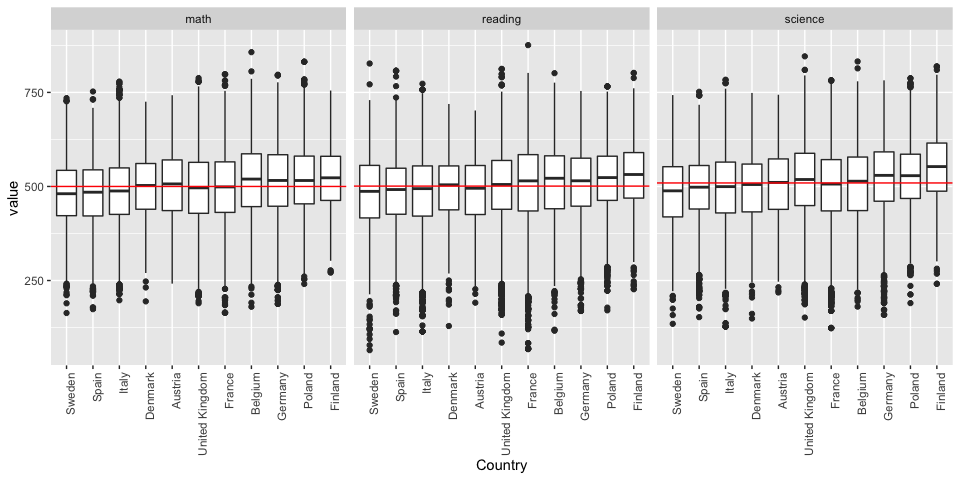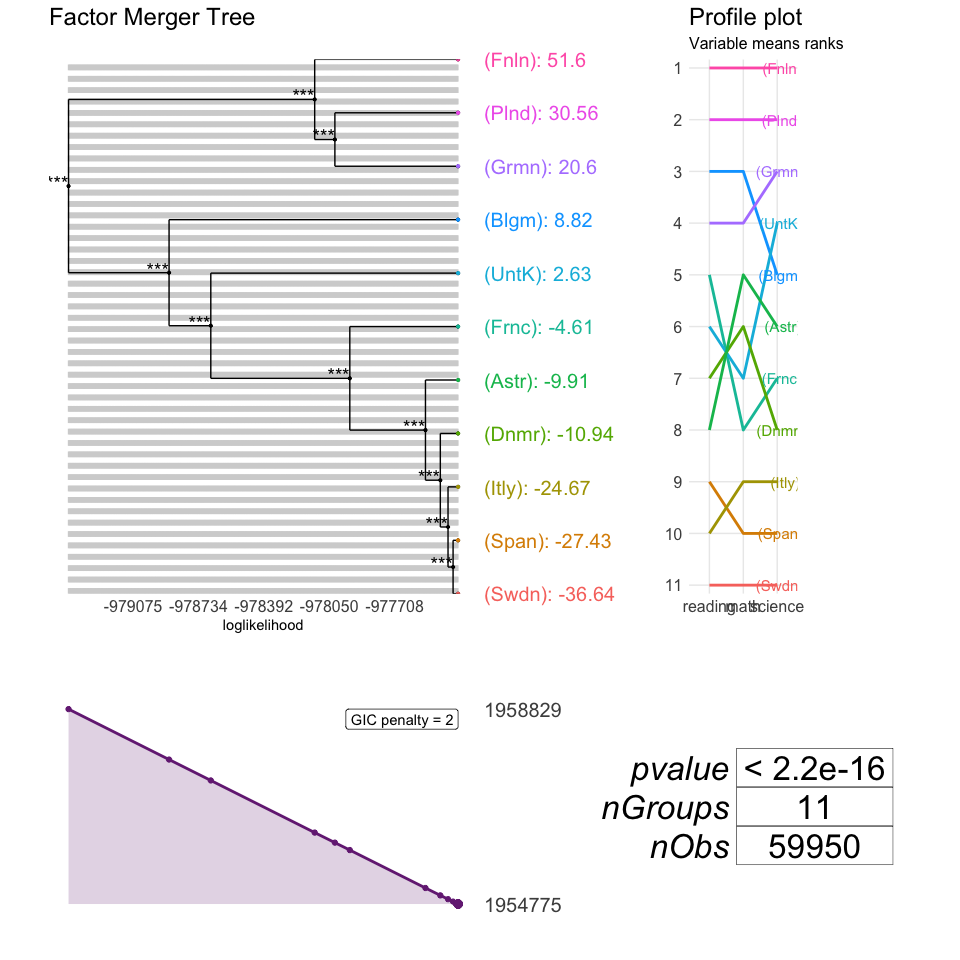# Libraries

library(factorMerger)
library(ggplot2)
library(dplyr)
library(reshape2)

data("pisa2012")

# Explore

pisa2012 %>% ggplot(aes(x = country)) + geom_bar() +
theme(axis.text.x = element_text(angle = 90, hjust = 1))meltedPisa <- pisa2012 %>% melt(na.rm = TRUE)
pisaResultsBySubject <-  meltedPisa %>%
ggplot(aes(x = reorder(country, value, FUN = median), y = value)) + geom_boxplot() +
facet_wrap(~variable) +
theme(axis.text.x = element_text(angle = 90, hjust = 1)) +
xlab("Country") 
pisaResultsBySubject +
geom_hline(data = meltedPisa %>% group_by(variable) %>% summarise(mean = mean(value)),
aes(yintercept = mean, group = variable), col = "red")TODO: Find countries significantly better, worse and not significantly different from global averages. Cluster countries into three groups.

# Run MANOVA

manova(cbind(math, reading, science) ~ country, pisa2012) %>% summary()
#>              Df   Pillai approx F num Df den Df    Pr(>F)
#> country      10 0.066994   136.91     30 179817 < 2.2e-16 ***
#> Residuals 59939
#> ---
#> Signif. codes:  0 '***' 0.001 '**' 0.01 '*' 0.05 '.' 0.1 ' ' 1

It seems that there exist some differences among countries included in PISA. Let’s find them!

# Factor Merger

Let’s now have a try using factorMerger for exploration.

It’s faster to use “fast-adaptive” or “fast-fixed” methods on a big dataset. They enable comparisons between neighbours only (neighbours are pairs of groups with close means).

pisaFMHClustEurope <- mergeFactors(response = pisa2012[,1:3],
factor = factor(pisa2012\$country),
method = "fast-fixed")

plot(pisaFMHClustEurope)